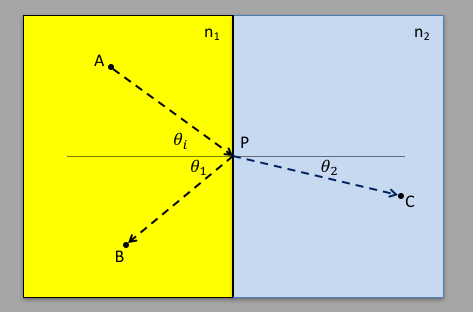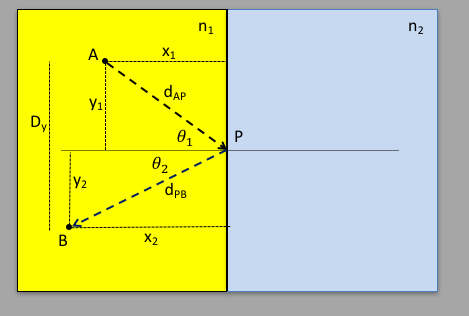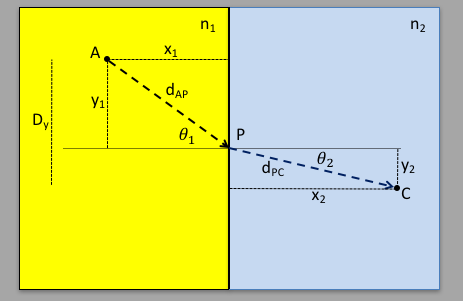(End of Page)

## Introduction

Back to top

In optics, we study visible light, and how it interacts with various materials classically. A very interesting aspect of this study throughout the history of optics involves whether light is a wave, or a particle (where we can think of light "rays"). By doing experiments that involve wave mechanics, like interference, refraction, diffraction, etc, we can easily see the wave nature of light. However, Isaac Newton led the group of natural philosophers who argued that light was not a wave, but instead a "ray", perhaps of particles, and pointed to rectilinear progapation as proof, arguing that a wave would propogate in all directions. The next section shows how we can use rectilinear progagation, with some dynamical principles, to understand phenomena such as reflection and refraction.

## Principle of Least Time

Back to top

Reflection and refection address what happens when light hits a surface at some incident angle $\theta_i$. In the figure below, the light ray starts at point A in the yellow region and travels to the interface with the blue region along the arrow at an angle $\theta_i$. Some of the light is then reflected at the interface and travels to point B at an angle $\theta_1$, and some goes through the interface and travels to point C along the ray in the blue region at an angle $\theta_2$. All angles are with respect to the normal to the interface.Here's where the physics starts: the velocity of the rays depends on the medium, which we can label as "medium $n$". We characterize this velocity in units of the velocity of light $c$, taking the ratio to form the "index of refraction": $$n=\frac{c}{v_n}\label{eindexrefraction}$$ Putting $c$ in the numerator means $n\ge 1$ always. Some interesting values for $n$:

 vacuum air water glass saphire diamond 1.0 1.0003 1.333 1.5 1.77 2.4

Light is an electromagnetic wave, generated by some source at some frequency $\nu$ propagating at some velocity $v$. In the vacuum, $v=c\equiv 3\times10^8$m/s. The wavelength of light is determined by the velocity in the medium, but the frequency is determined by the source. What determines the index of refraction is complex and has to do with how the light interacts with atoms and molecules in the medium, changing the velocity and the wavelength. But the frequency is the same in all mediums. Since the wavelength $\lambda$ and the frequency $\nu$ are related by $v=\lambda\nu$, then we have $$\lambda_n=\frac{v_n}{\nu}=\frac{c}{n\nu}=\frac{\lambda}{n}\label{elambdan}$$ where $\lambda$ is the wavelength in the vacuum where $v=c$.

What we want to do is to calculate the angles $\theta_1$ and $\theta_2$, using the ray properties of light, and that the velocity in the medium is a function of the medium. There are several approaches, but here we will use as our first principles the following theorem: the light will travel between any 2 points along a path that minimizes the total travel time. This is known as the principle of least time (and can be related to more complex concepts as the principle of least action).

Now that we know the velocities in the individual mediums, we can calculate the time $T_{AB}$ for the light to go from A to B (reflected) and $T_{AC}$ for the light to go from A to C (refracted).

#### Reflection

The figure below sets up the calculation for the case of reflection:Light starts at point A, and travels with velocity $v_1=c/n_1$ to point P and is reflected to point B. The distance from point A to P is $d_{AP}$ and from point P to B is $d_{PB}$. The total time it takes to get from A to B via P is: $$T_{AB} = \frac{d_{AP}}{v_1} + \frac{d_{PB}}{v_1}\nonumber$$

We can write $d_{AP}=\sqrt{x_1^2+y_1^2}$ and $d_{PB}=\sqrt{x_2^2+y_2^2}$. The vertical distance between the two points $D_Y$ is fixed, therefore $y_2=D_Y-y_1$. So we can write the total time $T_{AB}$ as: $$T_{AB} = \frac{\sqrt{x_1^2 + y_1^2}}{v_1} + \frac{\sqrt{x_2^2 + (D_Y-y_1)^2}}{v_1}\nonumber$$ We now have a total time $T_{AB}$ that is a function of a single parameter, $y_1$, so we find the extremem by setting the derivative to 0: $$\frac{dT_{AB}}{dy_1}=\frac{y_1}{v_1\sqrt{x_1^2 + y_1^2}} - \frac{D_Y-y_1}{v_1\sqrt{x_2^2 + (D_Y-y_1)^2}} =0\nonumber$$ The terms $v_1$ drop out, and we can write $y_1/sqrt{x_1^2 + y_1^2}=\sin\theta_1$ and $(D_Y-y_1)/sqrt{x_2^2 + (D_Y-y_1)^2}=\sin\theta_2$, which gives $\sin\theta_1=\sin\theta_2$, or equivalently $\theta_1=\theta_2$ which gives us the law of reflection: $$\theta_{reflected}=\theta_{incident}\label{ereflection}$$ As you can see, the velocity in the medium is irrelevant as far as reflection is concerned, since it never leaves the medium. The path of least (total) time is the path that has equal incident and reflected angles.

#### Refraction

The problem of refraction is attacked in the same way, however this problem has an interesting analogy. Imagine two people at the beach as in the figure below. A life guard is sitting on the beach at position A at some perpendicular distance from the water, watching the swimmers. At some position C in the water, one of the swimmers starts to cramp. The swimmer signals to the life guard, who runs to the beach, swims to point C, and pulls the drowning swimmer to safety. What path will the life guard take? Obviously, the path that takes the least time.

The velocity of the life guard is different in the different mediums: running is faster than swimming ($v_{run}\gt v_{swim}$). So going in a straight line from A to C ($\theta_1=\theta_2$) is not going to be in the least time. Perhaps running to a point directly perpendicular to the swimmer and swimming the shortest distance might be the path of least time ($\theta_2=0$). However, that maximizes $\theta_1$, and you can imagine that by changing the point of entry into the water (P) by a small amount towards A, the distance $d_{AP}$ will change by more than will the distance $d_{PC}$, making the total time smaller. So somewhere there has to be a sweet spot where the time is maximized.

In the simulation below, point P is set at some place between A and C both horizontally and more importantly, vertically. If you grab the circle at P with the mouse, you can move the point where the life guard enters the water, in the vertical direction. To the right, you will see the total transit time, taking into account the different velocities in the different medium, plotted in the vertical against the position $P(y)$, plotted along the horizontal. As you move P up and down, you can see where the minimum transit time is, and read off the two angles $\theta_1$ and $\theta_2$. What we want to do is calculate the relationship between those two angles $\theta_1$ and $\theta_2$.

 $n_1$ $n_2$

The same procedure applies to the refracted light which ends up at point C. The distances will be $d_{AP}$ and $d_{PC}$. For refraction we use the same definition for the velocity $v_n$, and calculate the total transit time between A and C, using the following diagram:$$T_{AC} = \frac{d_{AP}}{v_1} + \frac{d_{PC}}{v_2}\nonumber$$ where $nv_n=c/n$. We can write $d_{AC}=\sqrt{x_1^2 + y_1^2}$ and $d_{CP}=\sqrt{x_2^2 + y_2^2}$, and use the fact that $y_1+y_2=D_Y$, a fixed distance. The total time is then: $$T_{AC} = \frac{\sqrt{x_1^2 + y_1^2}}{v_1} + \frac{\sqrt{x_2^2 + (D_Y-y_1)^2}}{v_2}\nonumber$$ To find the extremen in $T_{AC}$, we set the derivate with respect to $y_1$ to zero and solve: $$\frac{dT_{AC}}{dy_1} = \frac{y_1}{v_1\sqrt{x_1^2 + y_1^2}} - \frac{D_y-y_1}{v_2\sqrt{x_2^2 + (D_Y-y_1)^2}}=0\nonumber$$ We can now use the fact that $$\sin\theta_1 = \frac{y_1}{\sqrt{x_1^2 + y_1^2}}$$ $$\sin\theta_2 = \frac{y_2}{\sqrt{x_2^2 + y_2^2}} = \frac{D_Y-y_1}{\sqrt{x_2^2 + (D_Y-y_1)^2}}\nonumber$$ to get the equation $$\frac{\sin\theta_1}{v_1}=\frac{\sin\theta_2}{v_2}\nonumber$$ or equivalently, using $v_1=c/n_1$ and $v_2=c/n_2$: $$n_1\sin\theta_1 = n_2\sin\theta_2\label{esnell}$$ This is called "Snell's Law".

In the figure below, you can use the mouse to grab point A, and move it around. The code will use Snell's law to calculate the refracted angle $\theta_2$ and draw the path inside medium that has index of refraction $n_2$. What you can see is that if light enters a region where the index of refraction is increasing, the refracted ray will be bent towards the normal.

 $n_1$ $n_2$

If you move point A to the blue region, or use the sliders and reverse the index of refraction in the 2 regions such that $n_1\gt n_2$, you can see that the ray path in region $2$ is bent away from the normal (it is the reverse of the situation where $n_2\gt n_1$). At some value of $\theta_i=\theta_C$, $\theta_2$ is along the inteface between the two regions, and any value of $\theta_i$ larger than that will cause the ray to be relfected ($\theta_2\gt \pi/2$). The angle $\theta_C$ is called Brewster's angle, and is that angle that causes total internal reflection: the light ray hitting the surface will be reflected, not refracted. This is what allows fiber optics to work: you introduce light that has a large angle relative to the normal to the surface (along the axial direction), and any light that hits the inside surface will be reflected back into the interior, staying in the fiber optic.

Copywrite Drew Baden, March 6, 2017